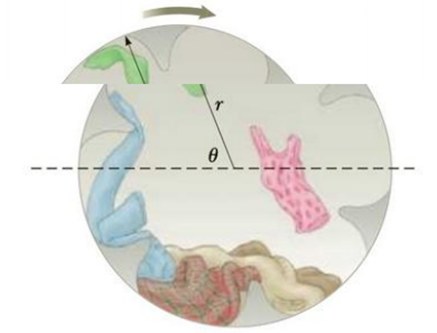Chapter 7, Problem 61AP

Chapter
Section
Textbook Problem

In a home laundry dryer, a cylindrical tub containing wet clothes is rotated steadily about a horizontal axis, as shown in Figure P7.61. So that the clothes will dry uniformly, they are made to tumble. The rate of rotation of the smooth-walled tub is chosen so that a small piece of cloth will lose contact with the tub when the cloth is at an angle of θ = 68.0° above the horizontal. If the radius of the tub is r = 0.330 m, what rate of revolution is needed in revolutions per second?Figure P7.61

To determine
The rate of revolutions per second of the tub.

Explanation

Given info: The small pieces of cloth will lose its contact with the tub when the cloth is at an angle of 68.0° above the horizontal. The radius of the tub is 0.330m .

Explanation:

When the radial component of the weight of the cloth exceeds the required centripetal force, the cloth will fall apart from the tub.

mgsinθmrω2

• m is the mass of the cloth
• g is the free fall acceleration
• θ is the angle between the cloth and the horizontal
• ω is the angular speed of the tub
• r is the radius of the tub

The cloth will fall apart when,

mgsinθ=mrω2

Re-arrangement for the expression for the angular speed,

ω=gsinθr

Substitute 9.80ms-2 for g , 0.330m for r , 68

Still sussing out bartleby?

Check out a sample textbook solution.

See a sample solution

The Solution to Your Study Problems

Bartleby provides explanations to thousands of textbook problems written by our experts, many with advanced degrees!

Get Started